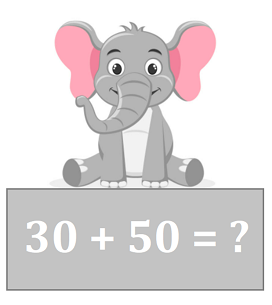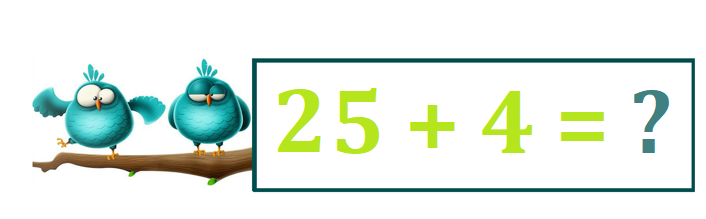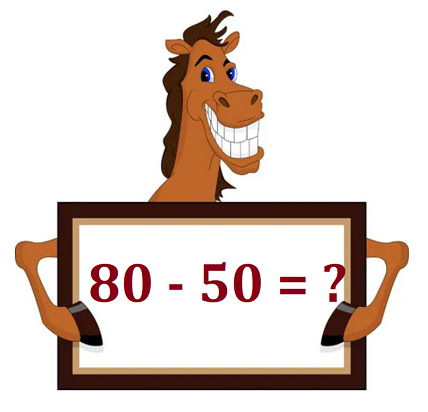# Math > Year 2

## Using number facts to 20 to add & subtract up to 100

Number facts: Number facts are the facts that help us to make the calculations easy.

Let’s learn some ways in which we can add or subtract numbers up to 100 using the facts up to 20.

Example 1:We will take the help of an easy fact to work out this question.

If we know that 3 + 5 = 8, then we can work out the sum of 30 and 50 as well.

3 has become ten times larger and 5 has become ten times larger, so the total (answer) will be ten times larger.

So, 30 + 50 = 80

Example 2:We will take the help of an easy fact to work out this question.

If we know that 15 + 4 = 19, then we can work out the sum of 25 and 4 as well.

We need to observe that one ten has been added to the 15, so, the total (answer) will also be increased by one ten.

25 + 4 = 29

Example 3:Explanation:

We will take the help of an easy fact to work out this question.

If we know that 5 + 3 = 8, then we can work out the subtraction of 50 from 80 as well.

5 has increased ten times and became 50 and 3 have increased ten times, which means the answer will also increase by ten times.

5 + 3 = 8

That means, 50 + 30 = 80

We can rewrite it in another way, as 80 - 50 = 30.

Let’s try some questions:

Question 1:

How can we work out 16 + 12?

Explanation:

We will take the help of an easy fact to work out this question.

If we know that 6 + 2 = 8, then we can work out the sum of 16 and 12 as well.

We need to observe that one ten has been added to the 6 and one ten has been added to 2, so, the total (answer) will also be increased by two tens.

16 + 12 = 28

Question 2:

How can we work out the operation "subtraction" using the numbers 90 and 20?

Explanation:

We know, 9 - 2 = 7
Then, 90 take away 20 will be 70.

//